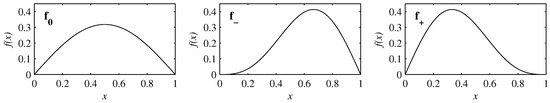Next Article in Journal
Statistical Mechanics-Based Schrödinger Treatment of Gravity

Metrics 0

## Export Article

Open AccessArticle

# Information Geometry of Spatially Periodic Stochastic Systems

1
Department of Applied Mathematics, University of Leeds, Leeds LS2 9JT, UK
2
School of Mathematics and Statistics, University of Sheffield, Sheffield S3 7RH, UK
*
Author to whom correspondence should be addressed.
Entropy 2019, 21(7), 681; https://doi.org/10.3390/e21070681
Received: 8 June 2019 / Revised: 4 July 2019 / Accepted: 10 July 2019 / Published: 12 July 2019
(This article belongs to the Special Issue Statistical Mechanics and Mathematical Physics)
|
PDF [590 KB, uploaded 12 July 2019]
|

# Abstract

We explore the effect of different spatially periodic, deterministic forces on the information geometry of stochastic processes. The three forces considered are $f 0 = sin ( π x ) / π$ and $f ± = sin ( π x ) / π ± sin ( 2 π x ) / 2 π$ , with $f -$ chosen to be particularly flat (locally cubic) at the equilibrium point $x = 0$ , and $f +$ particularly flat at the unstable fixed point $x = 1$ . We numerically solve the Fokker–Planck equation with an initial condition consisting of a periodically repeated Gaussian peak centred at $x = μ$ , with $μ$ in the range $[ 0 , 1 ]$ . The strength D of the stochastic noise is in the range $10 - 4$ $10 - 6$ . We study the details of how these initial conditions evolve toward the final equilibrium solutions and elucidate the important consequences of the interplay between an initial PDF and a force. For initial positions close to the equilibrium point $x = 0$ , the peaks largely maintain their shape while moving. In contrast, for initial positions sufficiently close to the unstable point $x = 1$ , there is a tendency for the peak to slump in place and broaden considerably before reconstituting itself at the equilibrium point. A consequence of this is that the information length $L ∞$ , the total number of statistically distinguishable states that the system evolves through, is smaller for initial positions closer to the unstable point than for more intermediate values. We find that $L ∞$ as a function of initial position $μ$ is qualitatively similar to the force, including the differences between $f 0 = sin ( π x ) / π$ and $f ± = sin ( π x ) / π ± sin ( 2 π x ) / 2 π$ , illustrating the value of information length as a useful diagnostic of the underlying force in the system. View Full-Text
FiguresFigure 1

This is an open access article distributed under the Creative Commons Attribution License which permits unrestricted use, distribution, and reproduction in any medium, provided the original work is properly cited (CC BY 4.0).MDPI and ACS Style

Hollerbach, R.; Kim, E.-J. Information Geometry of Spatially Periodic Stochastic Systems. Entropy 2019, 21, 681.

Note that from the first issue of 2016, MDPI journals use article numbers instead of page numbers. See further details here.

1